# Subnetting Practice Questions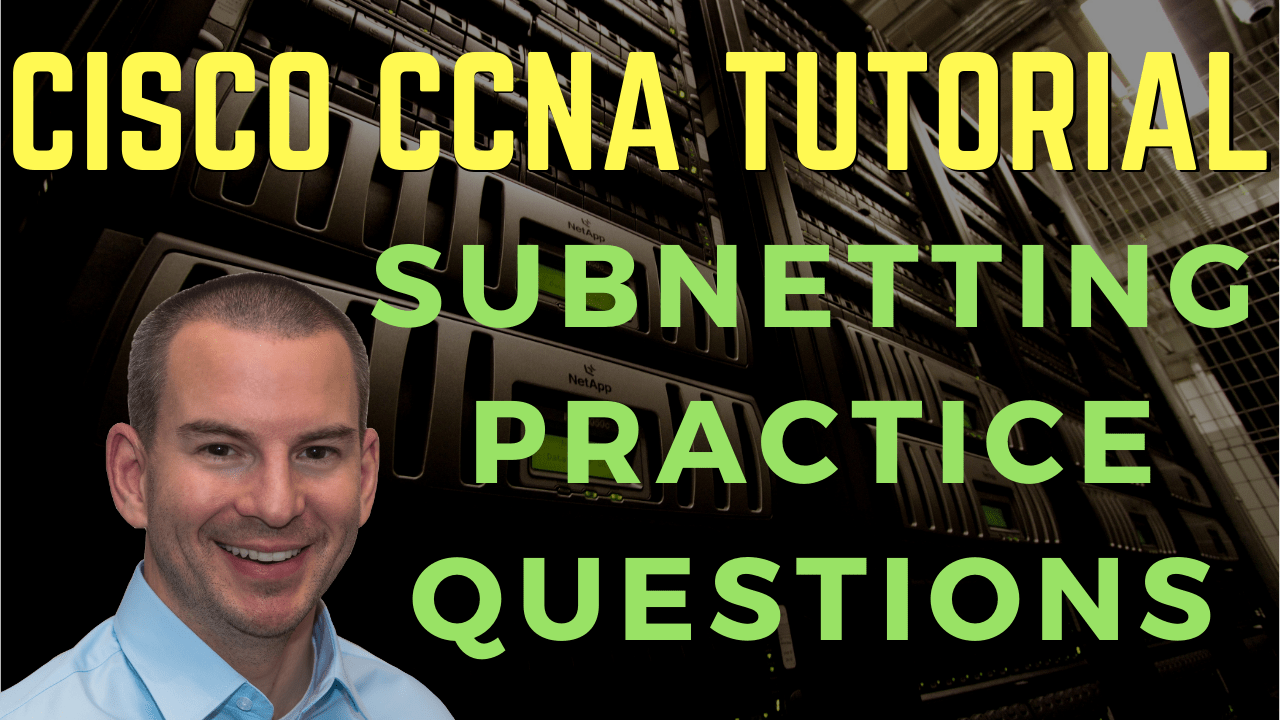In this Cisco CCNA training tutorial, we’re going to work through a subnetting practice question. Scroll down for the video and also text tutorials.

#### Subnetting Practice Questions Video Tutorial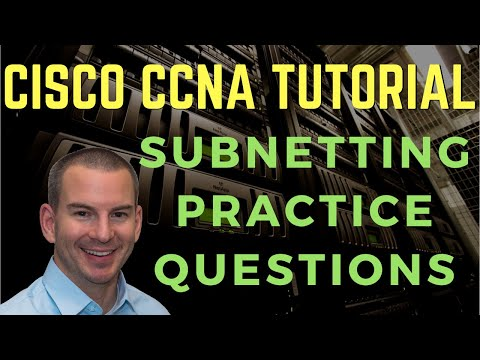### Jonathan Magpantay Lubag, Jr.I’m just letting you know that I passed the CCNA exam today and am now CCNA certified.  It’s all thanks to your course and Alphaprep that you endorse.

Jonathan Magpantay Lubag, Jr.

The question is:

A host has the IP address 198.22.45.173/26.

What are the network address, broadcast address, and valid host addresses for the IP subnet of which the host is a member of?

And the second part of the question - what is the subnet mask in dotted-decimal notation?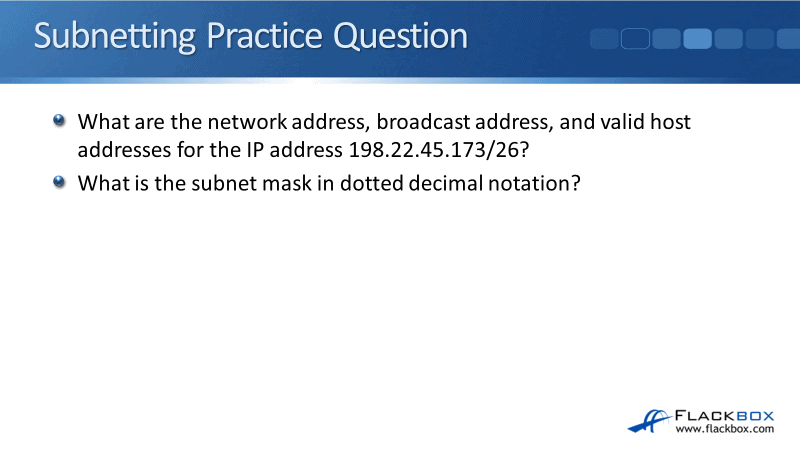Let's look at how we're going to figure out the answer to the question. We're going to do the second part first because it's easier and more logical to answer the question that way.

The second part of the question was, what is the subnet mask, /26, in dotted decimal? The way to do this when you're first learning subnetting is to write out the bit pattern at the top of a piece of paper.

Later on, you'll be able to do this in your head, but when you're first learning, it's way easier to write it all out. So, take a piece of paper, start at the top, and from right to left write out '1, 2, 4, 8, 16, 32, 64, 128' and put a dot in between each number. It will look like this: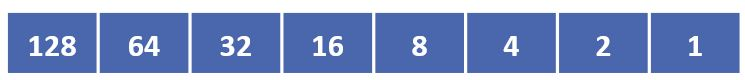Proceed to the next octet, until you've written down the bit pattern for the four octets in a subnet mask. Like this: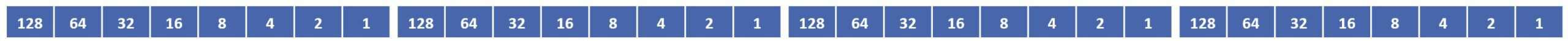The subnet mask in our example question is /26. Under your bit pattern at the top of the paper, write out 26 '1's underneath starting from the left side. Then, you can fill in the remainder with '0's for the host portion of the address: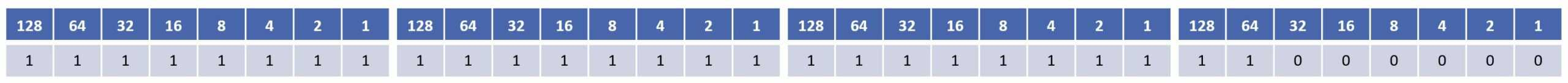Draw a line in after the 26 '1's to indicate the demarcation point between the network and host portions of the address (the network portion is to the left of the line, the host portion is to the right of it):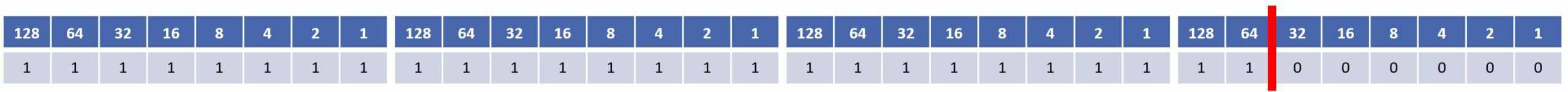Then we can just add up the '1's to find the subnet mask in dotted decimal notation.

The first octet is all '1's: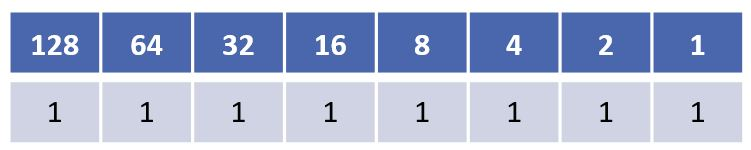128 + 64 + 32 + 16 + 8 + 4 + 2 + 1 = 255

255.

The second and third octets are also all '1's adding up to 255: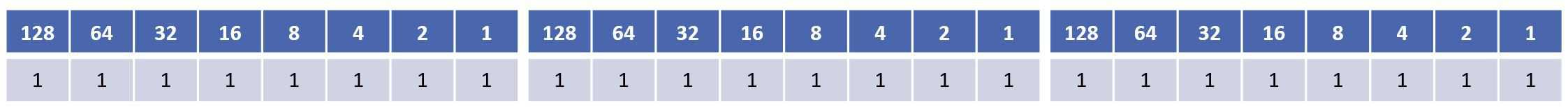255.255.255.

For the fourth octet, the '1's are under 128 and 64: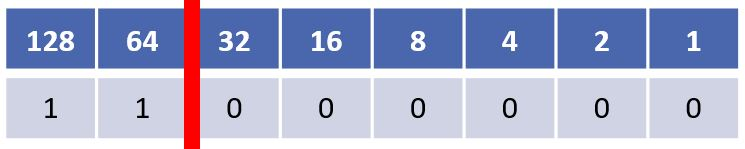128 + 64 = 192

Therefore, the subnet mask /26 in dotted decimal notation is 255.255.255.192.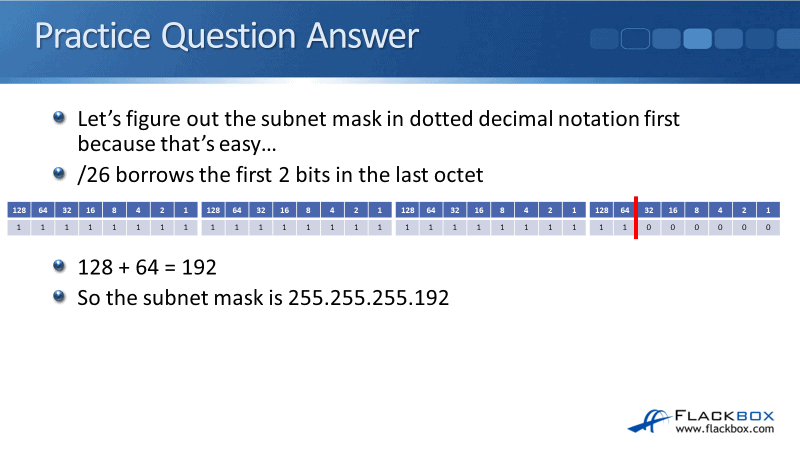Let's look at what the other part of the question was. What is the network address, the broadcast address, and a valid host address for the IP address, 198.22.45.173/26?

Again, we use our piece of paper and we're going to write out the IP address in dotted decimal notation now as well.

The IP address in our example question is 198.22.45.173. We start with the first octet which is '198'.

Starting from the '128' bit on the left, write down a '1' under the bit if it adds up to less than the number in the IP address. Let me explain.

The first octet is 198. 128 is less than 198, so we write down a '1' under the 128.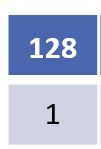The next bit after 128 is 64.

128 + 64 = 192

192 is less than 198 so we write a '1' under the 64.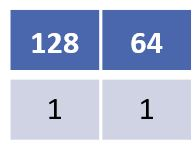The next bit after 64 is 32.

128 + 64 + 32 = 224

224 is more than 198, so we write a '0' (not a '1') under the 32.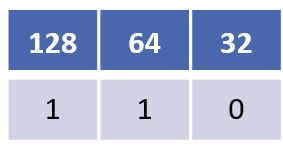The next bit after 32 is 16.

So far we have 128 + 64 = 192.

192 + 16 = 208

208 is more than 198, so we write a '0' under the 16.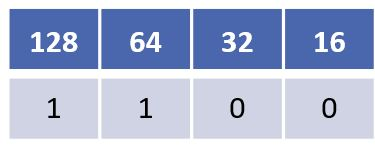The next bit after 16 is 8.

192 + 8 = 200

200 is more than 198, so we write a '0' under the 8.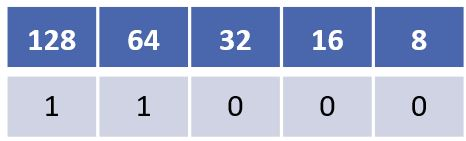The next bit after 8 is 4.

192 + 4 = 196

196 is less than 198 so we write a '1' under the 4.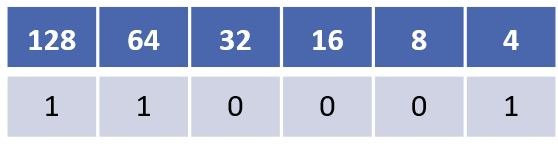The next bit after 4 is 2.

196 + 2 = 198

198 is exactly 198 so we write a '1' under the 2.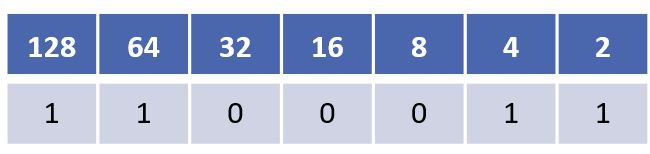We got to the exact number so we have finished calculating this octet. We can write a '0' down for all remaining bits, which is just the final '1' bit at the end in this case.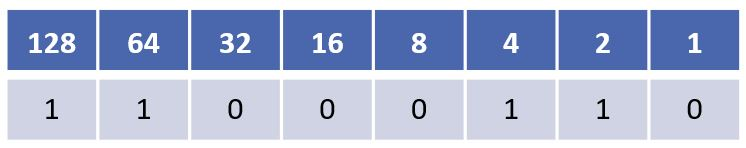Now that we've got it written down we can quickly add up the '1's to check our answer adds up to the '198' first octet in our 198.22.45.173 IP address.

128 + 64 + 4 + 2 = 198

We then repeat the process for the other 3 octets in order to write out the entire IP address 198.22.45.173 in dotted decimal notation: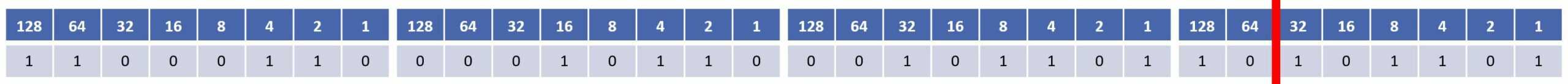We're working with a /26 subnet mask so the network portion of the address is the first 26 bits. The first 24 bits (first 3 octets) are 198.22.45, we can easily see that just by looking at the IP address.

Then we just count whatever it is in the 4th octet’s first two bits to get all 26 bits. I've got a 1 for 128 and a 0 for the 64, so that adds up to 128.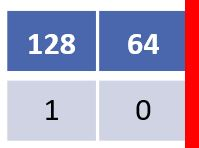So that's the first part of the question done. Next up is to figure out the broadcast addresses.

The broadcast address is the final IP address in this IP subnet, which is one less than the network address of the next IP subnet.

The network address of this IP subnet is 198.22.45.128 as we just calculated.

Looking at the line which demarcates the network and hosts portions of the address, we can see that it is after the 64.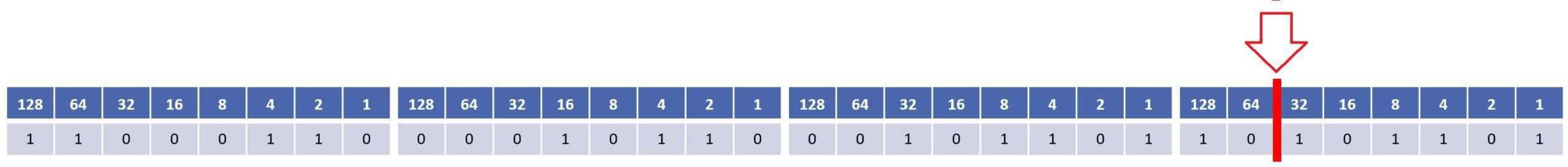So the IP subnets are going up in increments of 64, and as you can also see from the red line we are subnetting on the 4th octet.

This IP subnet is 198.22.45.128

To calculate the next IP subnet we add 64 to the octet we are subnetting on, which is the 4th octet in this case.

128 + 64 = 192

So the network address of the next IP subnet is 198.22.45.192

1 less than 198.22.45.192 is 198.22.45.191

That gives us 198.22.45.129 to 198.22.45.190.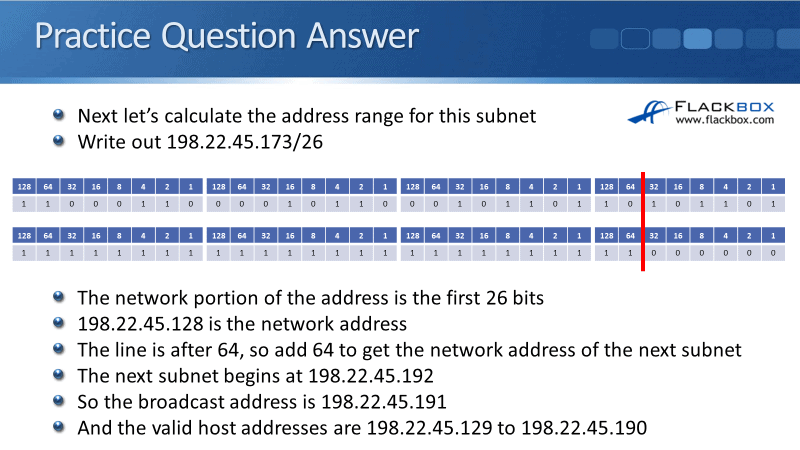A final important point to tell you here is, notice that all our calculations were done on the octet where the subnet line is. This example is a /26 so that is in the 4th octet. So we don't actually need to worry about the first three octets. They are always going to be unchanged as 198.22.45 in our example.

When working on a subnetting question, you only need to write out the octet that the subnetting is happening on. Because the other octets will always be unchanged, you don't have to write out the whole IP address in full every time.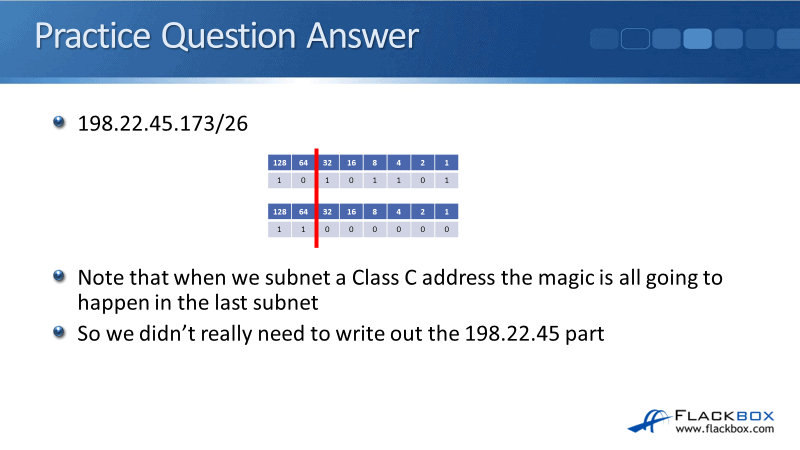#### Where to Get More Subnetting Practice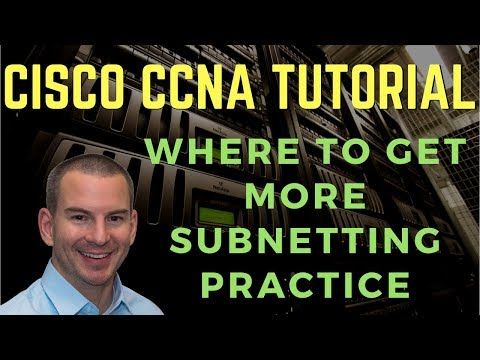If you're still not sure and want more practice, then a great resource for subnetting practice questions is subnettingpractice.com

The website has the same type of questions that you'll see on the CCNA exam. Every time you refresh the page, it will come up with a new question. It has variations on everything that’s subnetting related, so you should be confident that you can answer any of these questions after some practice.

I recommend you don't spend hours practicing subnetting in one sitting, that's no fun for anybody. To get ready for the exam, you can just pick your favourite practice site and spend five or 10 minutes a day working through a few problems.

If you do that for days or even weeks before the exam, you'll be fine when you get those subnetting questions. Another thing to tell you is that in the real world, people don't do subnetting in their head (if that wasn't obvious already).

What we do is we use an online subnet calculator, they're easy to find in Google.

Subnet calculators are quick and convenient to use when you need to do real world subnetting, and they're also very useful to check your answers when doing practice subnetting questions. Just plug in the numbers, and it will give you the answer.

Here's how we would verify our answers to this question at subnet-calculator.com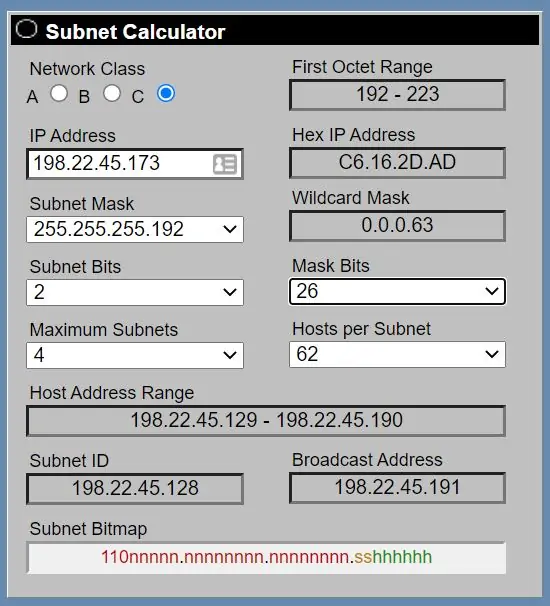Subnetting explained: https://study-ccna.com/subnetting-explained/

Click Here to get my Cisco CCNA Gold Bootcamp, the highest rated CCNA course online with a 4.8 star rating from over 20,000 public reviews.

### Libby TeofiloText by Libby Teofilo, Technical Writer at www.flackbox.com

With a mission to spread network awareness through writing, Libby consistently immerses herself into the unrelenting process of knowledge acquisition and dissemination. If not engrossed in technology, you might see her with a book in one hand and a coffee in the other.